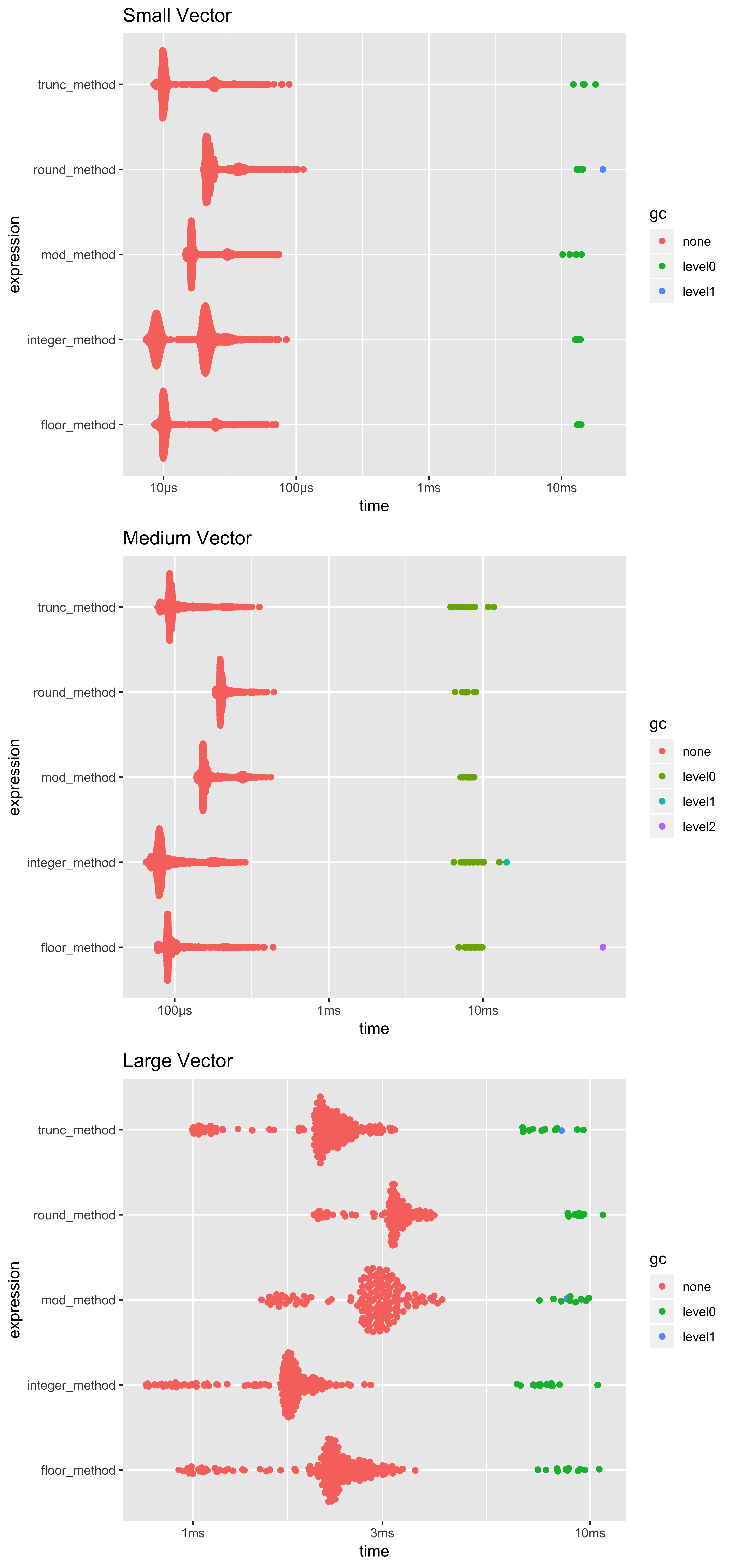# Testing Equality of Multiple Expressions

Tyler Littlefield · 2018/04/03 · 3 minute read

Credit to BrodieG: https://stackoverflow.com/questions/27325005

This post is essentially an extension of a previous post I wrote. The only addition is a cool idea for testing near equality of outputs. I’m calling this an “equality matrix”, a matrix of methods that displays whether or not they are equal to each other. One use case is benchmarking. As we benchmark multiple solutions to a single problem, testing whether or not the outputs are equal becomes more time consuming as the `all.equal` function only takes two solutions at a time.

Consider the following problem: extract all numbers in a vector that have a non zero value after the decimal.

We could do this a few ways:

``````# vector
x <- c(0.0, 0.5, 1.000, 1.5, 1.6, 1.7, 1.75, 2.0, 2.4, 2.5, 3.0, 74.0)

# create objects for testing equality of output
integer_method <- x[as.integer(x) != x]
trunc_method <- x[trunc(x) != x]
round_method <- x[round(x) != x]
mod_method <- x[x %% 1 != 0]
floor_method <- x[floor(x) != x]``````

Now instead of testing every combination to make sure the outputs are equal we can create a matrix that tests all possible combinations at once:

``````# create an equality matrix
methods_vec <- c("integer_method", "trunc_method", "round_method", "mod_method", "floor_method")
objs <- mget(methods_vec)
outer(objs, objs, Vectorize(all.equal))
#>                integer_method trunc_method round_method mod_method floor_method
#> integer_method           TRUE         TRUE         TRUE       TRUE         TRUE
#> trunc_method             TRUE         TRUE         TRUE       TRUE         TRUE
#> round_method             TRUE         TRUE         TRUE       TRUE         TRUE
#> mod_method               TRUE         TRUE         TRUE       TRUE         TRUE
#> floor_method             TRUE         TRUE         TRUE       TRUE         TRUE``````

That’s it! With just a few lines of code we can test the equality of multiple solutions and print the result nicely. For fun, let’s benchmark :smile:

If you’ve read my previous post on benchmarking, this should all be familiar. First we load the necessary libraries and then we create vectors of different size to test how each solution handles small, medium, and large data:

``````library(ggplot2)   # plotting
library(patchwork) # plot multiple plots
library(dplyr)     # using this for pipe functionality

# create vectors of different sizes
x <- c(0.0, 0.5, 1.000, 1.5, 1.6, 1.7, 1.75, 2.0, 2.4, 2.5, 3.0, 74.0)
xs <- rep(x, 1e2)
xm <- rep(x, 1e3)
xl <- rep(x, 1e4)``````

Now we benchmark:

``````i <- list(xs, xm, xl)

bench_all <- function(i) {
bench::mark(
integer_method = i[as.integer(i) != i],
trunc_method = i[trunc(i) != i],
round_method = i[round(i) != i],
mod_method = i[i %% 1 != 0],
floor_method = i[floor(i) != i]
)
}

results <- lapply(i, bench_all)``````

Then create the plot:

``````p1 <- autoplot(results[]) +
labs(title = "Small Vector")

p2 <- autoplot(results[]) +
labs(title = "Medium Vector")

p3 <- autoplot(results[]) +
labs(title = "Large Vector")

p1 / p2 / p3 ``````